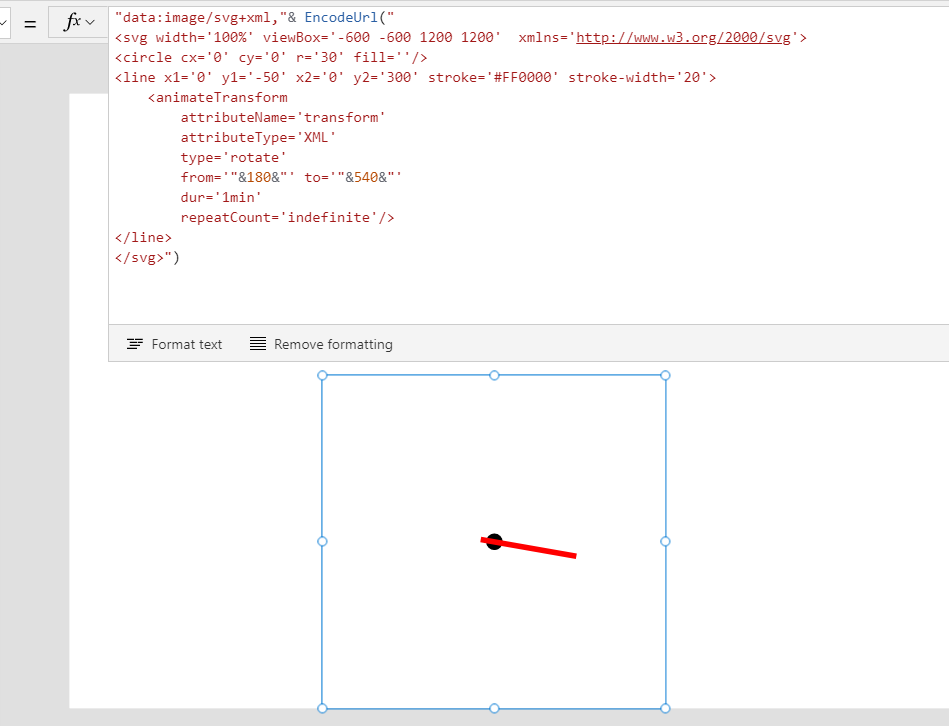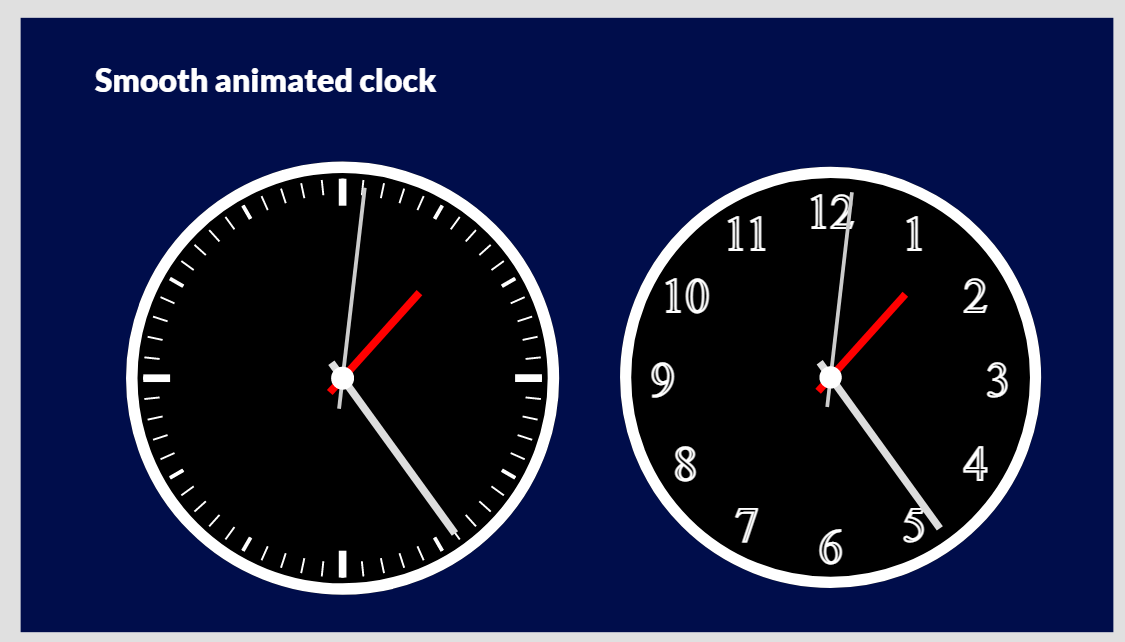# Smoothly animated clock without Timer control

So far, I saw many app makers made animated clock leveraging repeating Timer and some variable to update HTML/SVG code.

The animated clock in above Tweet is not using Timer control and works in edit mode (Power Apps studio) as well.

# How does it work??

The secret of this clock is use of "animateTransform" element in SVG.

This allows to move SVG elements without any external input - Timer event, which is applied to Hour/Minute/Second hands rotation.Key attribute of animateTransform here is dur (duration), these "Hand" rotate 360 degree clockwise in specified duration.

If you set dur = 1min, it will be Second hand, dur = 60min will be Minute hand, and dur = 24h, it will be Hour hand.

# Initialize Clock

To initialize clock hands, it requires to convert time value (Hour, Min, Sec) to degrees of each hands.

It is achieved by following expression:

```Set(degH,Value(Mod(Hour(Now())+Minute(Now())/60,12))/12*360);
Set(degM,Value(Minute(Now())+Second(Now())/60)/60*360);
Set(degS,Value(Second(Now()))/60*360);```

In my sample app, those expression is in OnVisible property of start screen.

# Sample code

You can download sample app from Community app gallery or copy n paste expressions to Image control.

Image1.Image

```"data:image/svg+xml,"& EncodeUrl("<svg width='100%' viewBox='-600 -600 1200 1200'  xmlns='http://www.w3.org/2000/svg'>

<circle cx='0' cy='0' r='550' fill='black' stroke='#000' stroke-width='30'/>
"&Concat(Sequence(60,0),"<line x1='0' y1='-520' x2='0' y2='-480' stroke='#FFF' stroke-width='5' transform='rotate("&6*Value&")'/>")&"
"&Concat(Sequence(12,0),"<line x1='0' y1='-520' x2='0' y2='-480' stroke='#FFF' stroke-width='10' transform='rotate("&30*Value&")'/>")&"
"&Concat(Sequence(4,0),"<line x1='0' y1='-520' x2='0' y2='-450' stroke='#FFF' stroke-width='20' transform='rotate("&90*Value&")'/>")&"
<circle cx='0' cy='0' r='550' fill='transparent' stroke='#FFF' stroke-width='30'/>
<line x1='0' y1='-50' x2='0' y2='300' stroke='#FF0000' stroke-width='20'>
<animateTransform
attributeName='transform'
attributeType='XML'
type='rotate'
from='"&degH+180&"' to='"&degH+540&"'
dur='12h'
repeatCount='indefinite'/>
</line>
<line x1='0' y1='500' x2='0' y2='-50' stroke='#DDD' stroke-width='20'>
<animateTransform
attributeName='transform'
attributeType='XML'
type='rotate'
from='"&degM+180&"' to='"&degM+540&"'
dur='60min'
repeatCount='indefinite'/>
</line>
<line x1='0' y1='500' x2='0' y2='-80' stroke='#CCC' stroke-width='10'>
<animateTransform
attributeName='transform'
attributeType='XML'
type='rotate'
from='"&degS+180&"' to='"&degS+540&"'
dur='60s'
repeatCount='indefinite'/>
</line>
<circle cx='0' cy='0' r='30' fill='#FFF'/>
</svg>")```

Screen1.OnVisible

```Set(degH,Value(Mod(Hour(Now())+Minute(Now())/60,12))/12*360);
Set(degM,Value(Minute(Now())+Second(Now())/60)/60*360);
Set(degS,Value(Second(Now()))/60*360);```